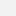# Humboldt-Universität zu Berlin - Statistik

Numerical Introductory Course (SE)

## Numerical Introductory Course (SE)Kategorie
Master
Lehrende(r)
S. Borak

# Description

After introducing basic terms and theorems, the seminar Numerical Introductory Course will deal with numerical methods and their applications in statistics and finance. Examples of implementation will be shown.

# Course Outline

After introducing basic terms and theorems, the course will deal with numerical methods and their applications in statistics and finance. Examples of implementation will be shown. The course will cover the following topics: Finite, iterative and gradient methods for linear systems and matrix inversion, matrix factorization, eigenvalues and eigenvectors, interpolation, numerical differentiation and integration, solving non-linear equations and their systems, unconstrained optimization and constrained optimization, random numbers generation and Monte Carlo.#### Topics for presentation:

• Computer arithmetic and inaccuracies
• Random number generation
• Finite algorithms for systems of linear equations
• Iterative algorithms for systems of linear equations
• Nonlinear equations (one-dimensional case)
• Gram-Schmidt orthogonalization
• Curve fitting II (least squares)
• Nonlinear equations (multidimensional case)
• Numerical differentiation
• Numerical integration
• Integer programming
• Optimization I (stopping criteria, 1D methods)
• Optimization II (multidimensional problems)
• Optimization III (constrained problems)
• Eigenvalues and eigenvectors
• Sorting algorithms
• Matrix decompositions
• Computing quantiles by bisection
• Fast Fourier Transform

# Literature

• Benko, M., Cizkova L., Härdle W. (2003), Numerical Methods in Statistics, MD*Booklet
• Gentle, J.E. (1998), Numerical Linear Algebra for Applications in Statistics, Springer Verlag, New York
• Gentle, J.E. (1998), Random Number Generation and Monte Carlo Methods, Springer Verlag, New York
• Huet, S. et al (1996), Statistical Tools for Nonlinear Regression, Springer Verlag, New York
• Lange, K. (1999), Numerical Analysis for Statisticians, Springer Verlag, New York
• Monahan, J.F. (2001), Numerical Methods of Statistics, Cambridge University Press, Cambridge
• Press, et al (1992), Numerical Recipes in C: The Art of Scientific Computing (2nd edition), Cambridge University Press, Cambridge
• Numerical Recipes - Books online at http://www.library.cornell.edu/nr/bookcpdf.html
• Woodford, C., Phillips, C. (1997), Numerical Methods with Worked Examples, Chapman & Hall, London
• Seydel, R., (2003), Tools for Computational Finance, Springer, Berlin
• Cizkova, L., Numerical Optimization Methods in Econometrics, in: Rodriguez Poo, J. M., Computer-Aided Introduction to Econometrics; electronic version
• An Introduction to LaTeX, incl. a link to "The (Not So) Short Introduction to LaTeX2e" in many languages.